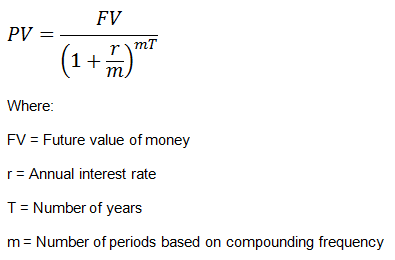# Present Value of a Single Cash Flow

Present value of a single cash flow refers to how much a single cash flow in the future will be worth today. The present value is calculated by discounting the future cash flow for the given time period at a specified discount rate.

The formula for calculating future value is:Example

Calculate the present value (FV) of a payment of $500 to be received after 3 years assuming a discount rate of 6% compounded semi-annually. FV = 500/((1+6%/2)^(2*3)) =$418.74

We can also solve this problem using the calculator as follows:

In our above example, enter FV = 500, change P/Y = 2 (semi-annual compounding), I/Y = 6, N = 6.

Then press CPT > PV. We get:

FV = 418.74